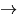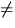## Definition of Word Accuracy in Japanese

• Definition of words depends on morphological analyzerChaSen
• Segmentation is still non-deterministic
(Ex.) $B$H$b(B + $B$K(B''$B$H$b$K(B''Align after concatenating compounds • Notation (use of Kanji/Kana) is also arbitrary (Ex.) $B==Fs(B''12''
(Ex.) $B0l?M(B''$B$R$H$j(B'' But $BA:n(B''`\$BA\::(B''Define accuracy in both Kanji-basis & Kana-basisNext: Acoustic Model Evaluation Up: Performance Evaluation Previous: Experimental Condition
Tatsuya Kawahara
5/31/2000